• In previous videos, we talked about the pipeline, where we put the videos through many segment of ML process.
• This video will focus mainly on what called Sliding Windows Classifier.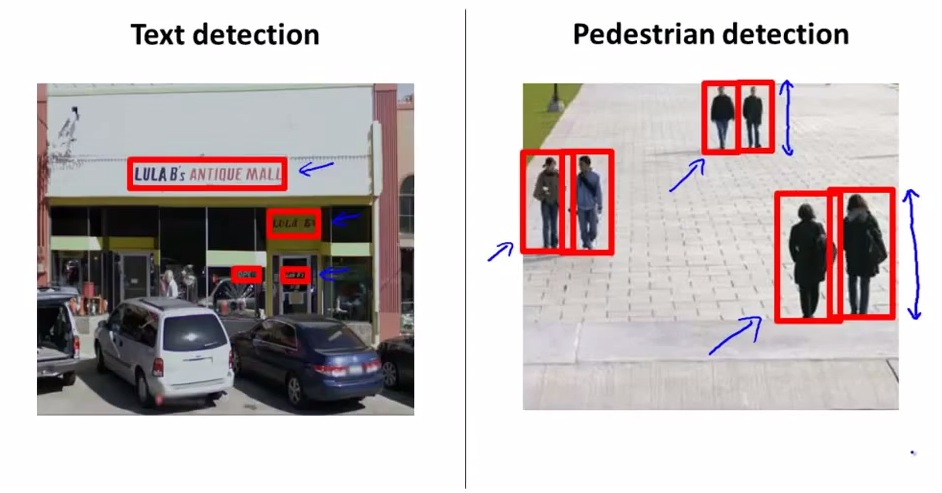• In the text detection, the problem in Computer Vision is a bit odd as the rectangle to recognize the characters within the image is all different
• Pedestrian detection will be used to explain more, as will go back to text detection after we get a better intuition.
• The pedestrian here have same aspect ratio, eventhough some are further or closer to the camera.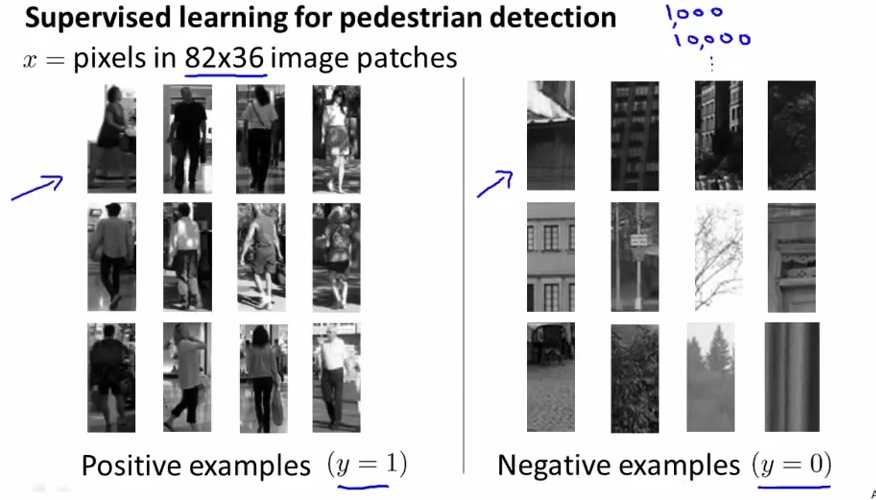• Here we have training set of our pedestrian(y=1) or not(y==0). This is the problem of supervised learning with neural networks. We will classify between these two label sets.
• We choose the pixels rectangle as stated.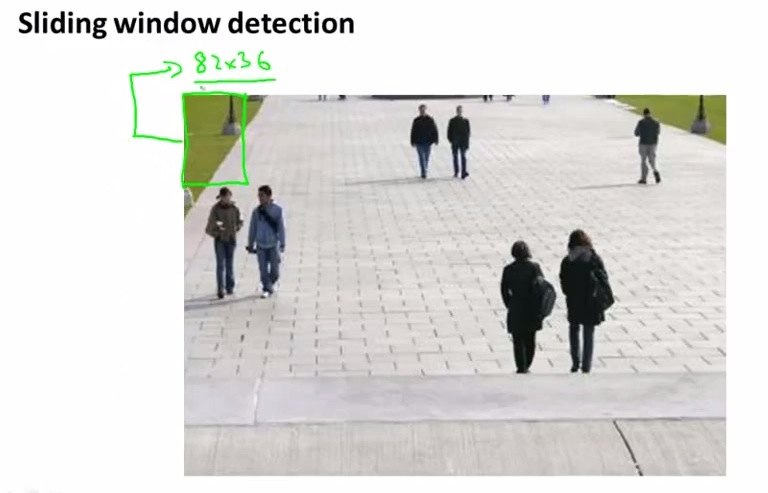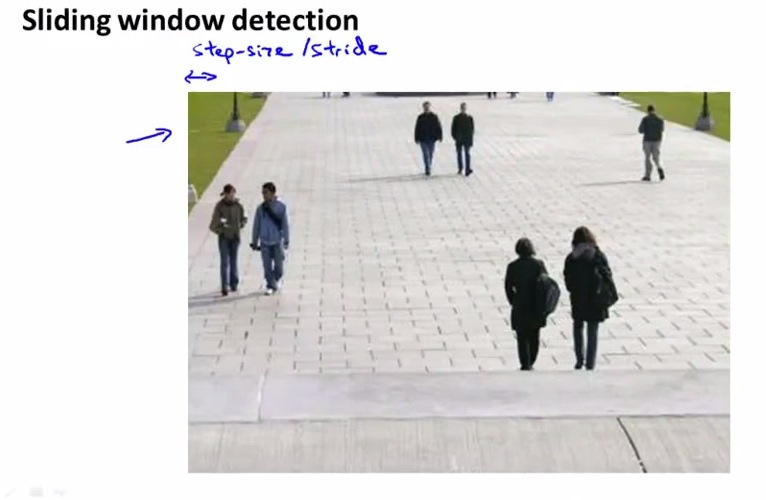• Now we want to scan all the pixels in the photo by iterating the rectangle
• It best with sliding step_size by 1 pixel at the time. But in result, will be computationally expensive
• From 82x36, we may want to slide 0.25 or 0.5 of the rectangle and iterating over to the bottom
• Having all that, we move to the bigger rectangle but still maintaining the aspect ratio. Using slightly bigger rectangle over and over again. Move to a bigger rectangle than before and keep doing it until the biggest rectangle to the bottom.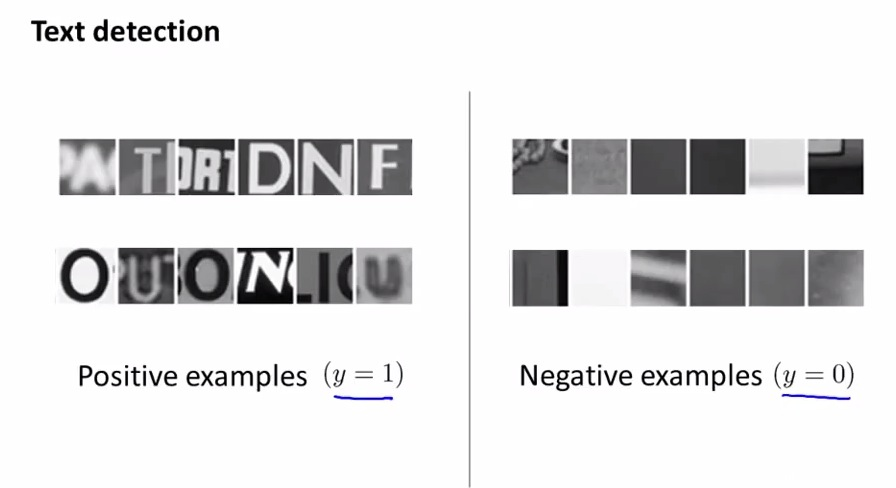• Now let's go back to text detection examples
• Here's we do all the step as earlier, where we have all training set of postive examples patch to ML problem, and negative examples also patch to ML problem.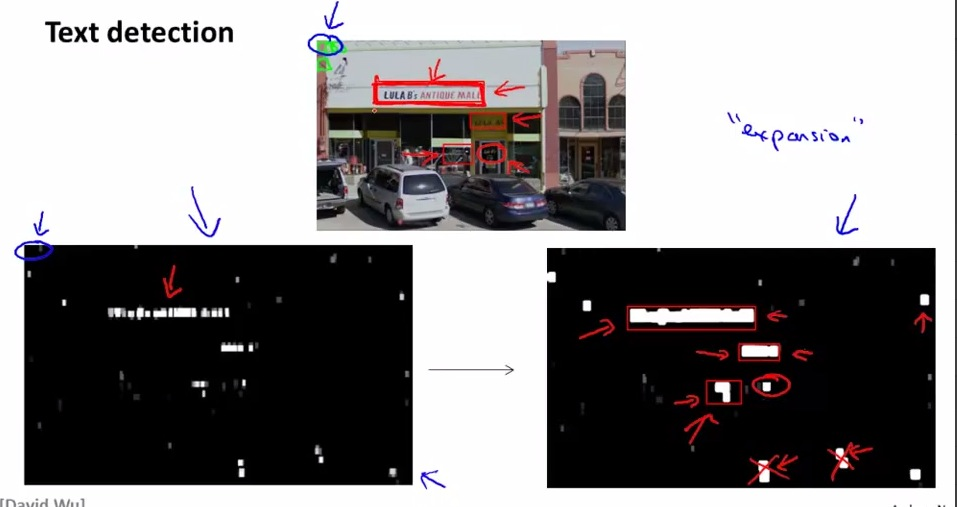• So for the pipeline of text detection here's what we do
• We are going to make rectangle that containing the characters
• Figure on the bottom left shown as the algorithm's performance in predicting the text.
• White shows high probability of there's a text in it, black shows none, gray show's there's might be a text in the photo.
• Next do what called "Expansion operator". We're going to border the white even more. Mathematically ways, if there's another white pixels in range of 5-8 pixels from the white pixels, then color white all of them. This way we're going to get definite bounding box white in all of the photos.
• Then, rule out the aspect ratio that is odd. In this example, text can't be bigger vertical than its horizontal size.
• Having all that, we still missing the writing in the transparant door that circle red in the example. It occurs because the text isn't clear enough and written in the transparent door.
• And that's the step of sliding window text detectioni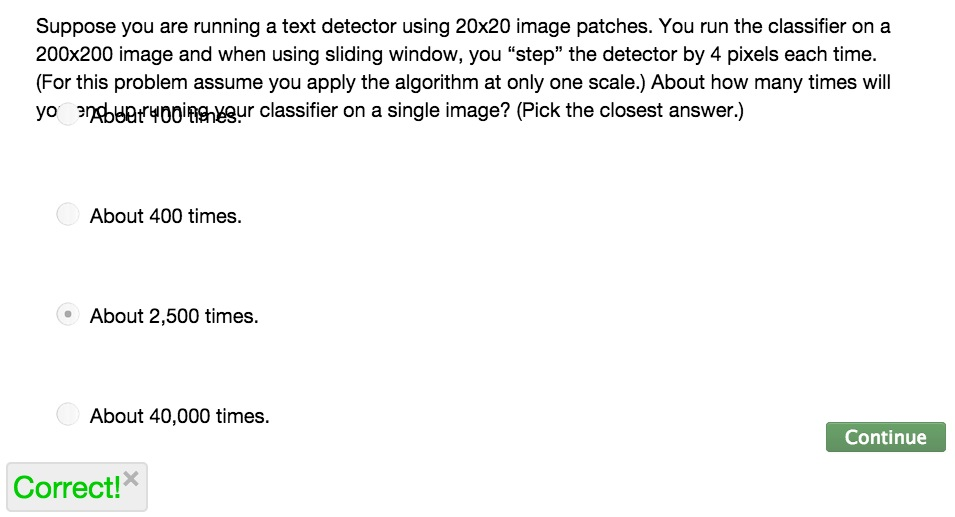• In the quiz we may want to forget the image patches size. And focus on the size_step. Because we detects 4 step_size on the total 200x200. so we have 50x50 step, which equals  2500 times.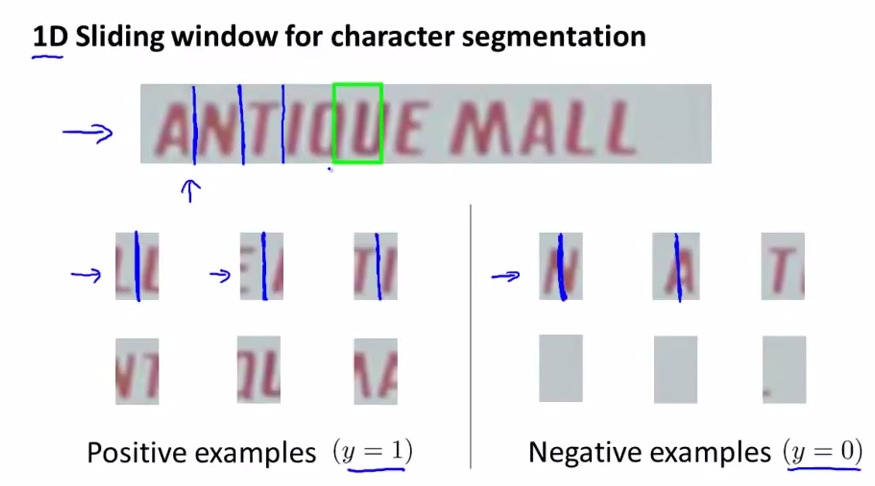• Now we will pursuit on the next step, character segmentation
• Here we're doing sliding window again, this time 1D, only one row from left to right.
• Again, we give our positive example and negative example
• So this is other machine learning problem, so we want to make another learning algorithm
• we give problem where positive examples is when we can split the photo in the middle, and confirm there's to character if we split. And negative example where's we can't split (either full one character, or no  character at all)
• This way we can segment the character correctly in the photo.
• Begin by iterating, if there's a split, put a blue line(or split it directly), if it doesn't then just move on to the next sliding window iteration.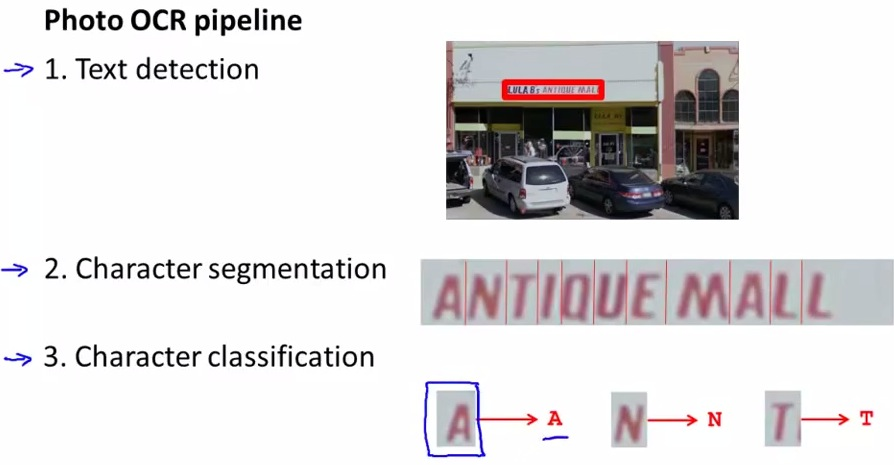• So that's all the step
• We using sliding windows on step one and two. First step sliding window to detect text within the images. Second step sliding window to segment characters within the image
• Third step is as we familiar with earlier either with using supervised learning or neural networks, with 26 characters or 36 characters (along with the digits)
• Next, still using Photo OCR to introduce other techniques in machine learning to solve similar problems in OCR